This post is based on problems 2.10 and 2.11 in, “Heard on the Street” by Timothy Falcon Crack. I was asked how to price a digital option in a job interview - and had no idea what to do!

# European Call Options

A European call option is the right to buy an asset at the strike price, $K$, on the option’s expiration date, $T$. A call is only worth exercising (using) if the underlying price, $S$, is greater than $K$ at $T$, as the payoff from exercising is $S-K$. The plot below shows the value of a call option, as a function of the underlying asset’s price, with $K=100$: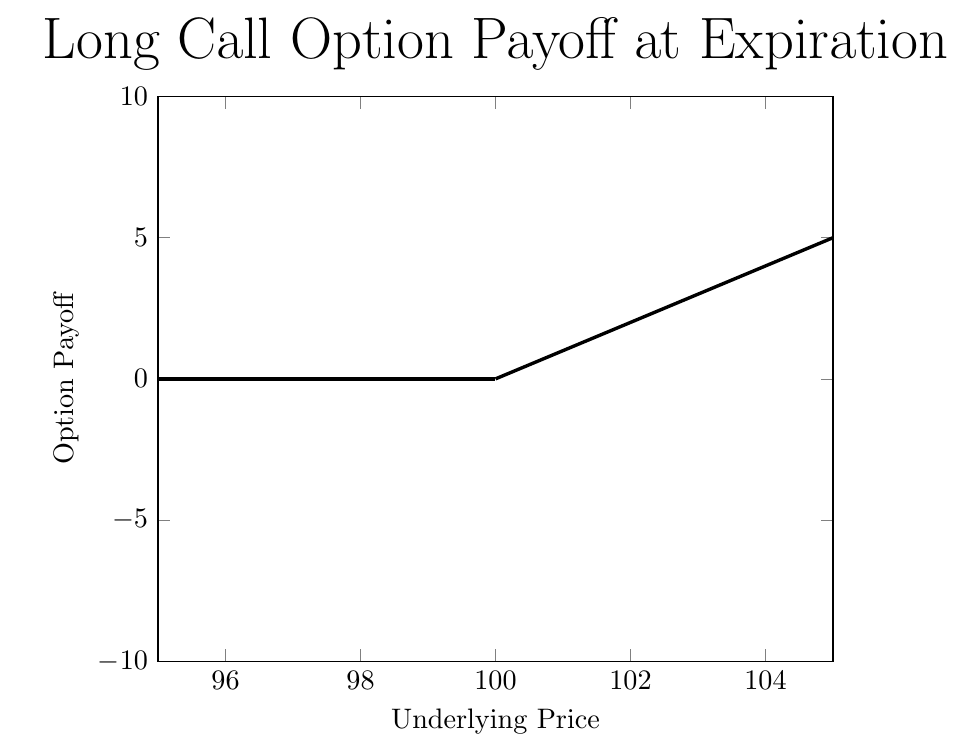Selling a call option with a strike $K=100$ earns you the call’s price, $c$, today, but your payoff will be decreasing in the underlying price: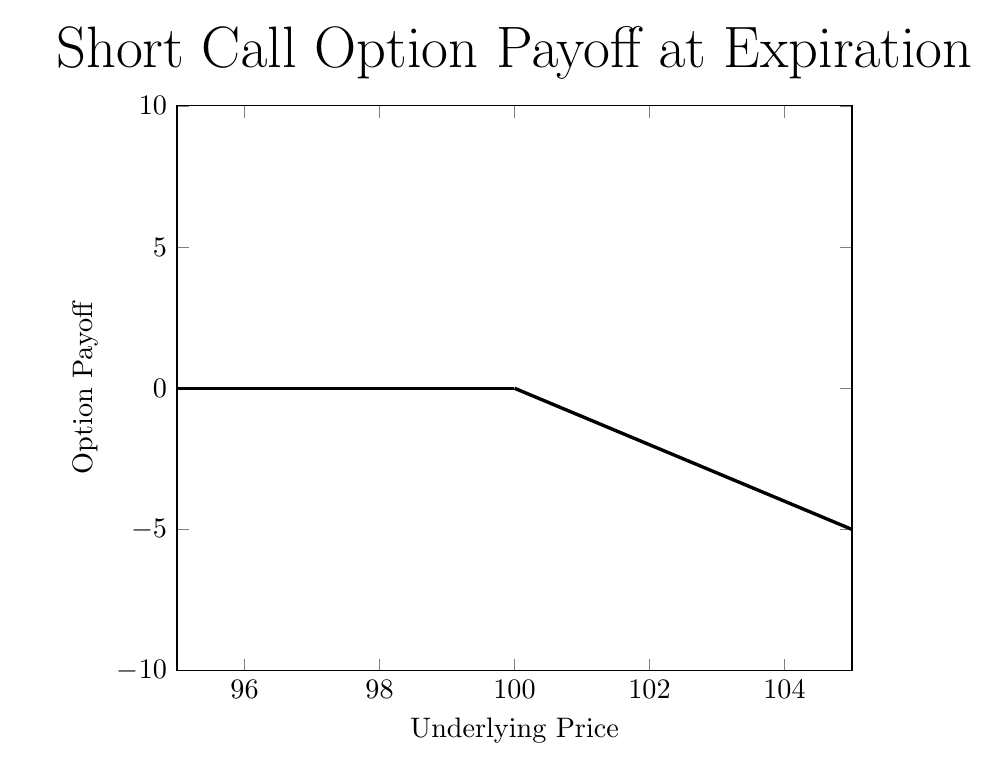# Digital Call Options

A digital call option with $K=100$ is similar - it pays off one dollar if $S\geq100$ at expiration, and pays off zero otherwise:Suppose you have a model for pricing regular call options. If you’re using Black-Scholes the price of the call, $c$, is a function of $K$, $S$, time to expiration $T-t$, the volatility of the underlying asset $\sigma$, and the risk free rate $r$: \begin{equation} c=F(K,S,T-t,\sigma,r) \end{equation} Now - suppose the model is correct. How can you use $F(K,\cdot)$ to price the digital option?

# Replicating the Digital Option

The trick is to replicate the digital option’s payoff with regular calls. As a starting point, consider buying a call with $K=100$ and selling a call with $K=101$: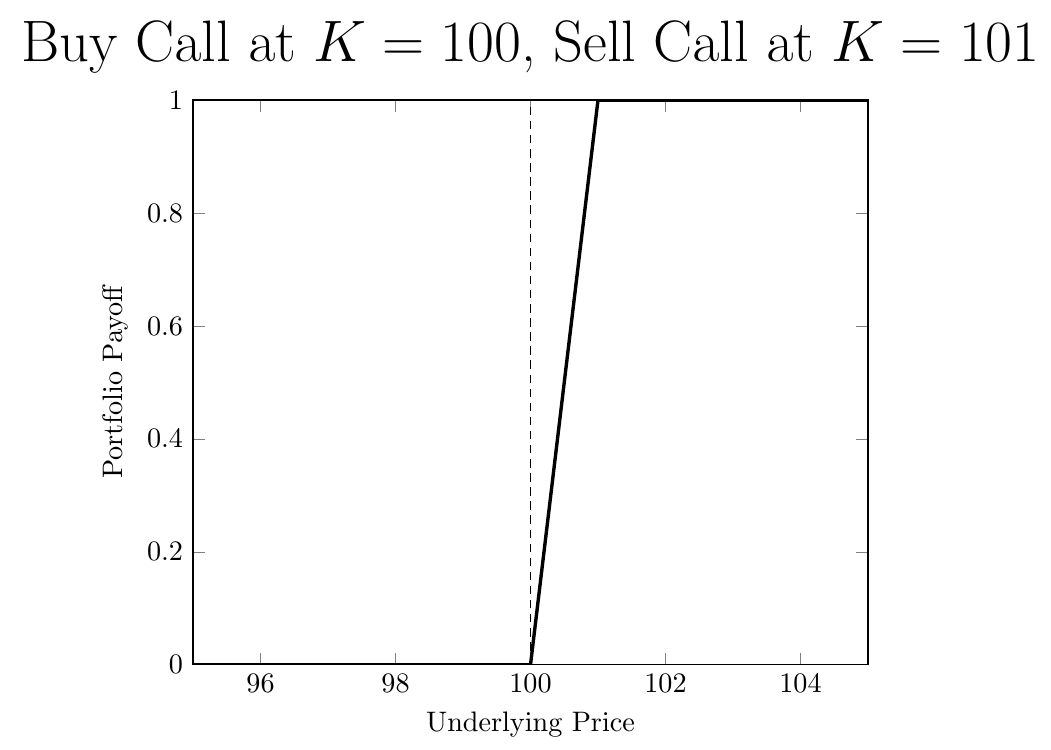This is close to the digital option, but not exactly right. We want to make the slope at 100 steeper, so we need to buy more options. This is because a call’s payoff increases one-for-one with the underlying once the option is in the money, so with one option you are stuck with a slope of one.
Consider buying two calls with $K=100$ and selling two calls at $K=100.5$: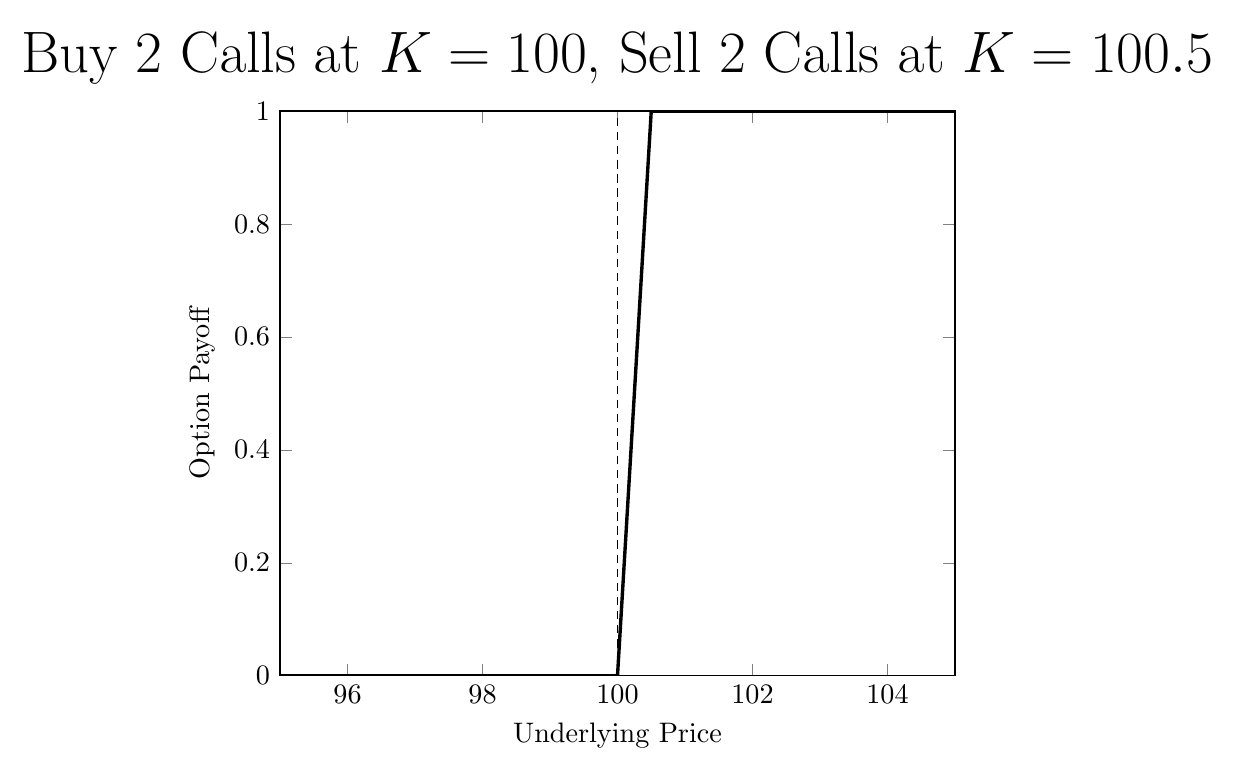As opposed to a slope of 1 between 100 and 101, now we have a slope of two between 100 and 100.5.

Generalizing this idea - consider a number $\epsilon>0$. To get a slope of $\frac{1}{\epsilon}$, you buy $\frac{1}{\epsilon}$ calls at $K=100$ and you sell $\frac{1}{\epsilon}$ calls at $K=100+\epsilon$. Here’s what it looks like for $\epsilon=\frac{1}{10}$: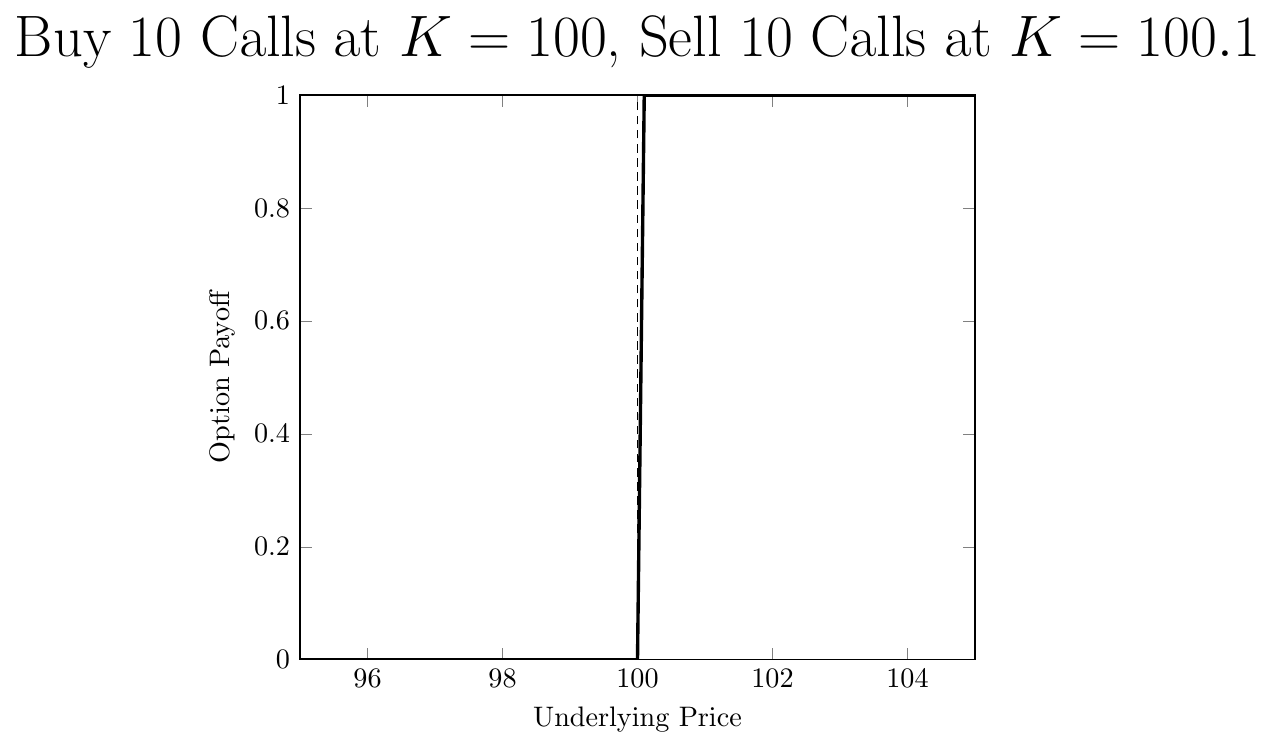Given that the slope is $\frac{1}{\epsilon}$, to get an infinite slope, we take the limit as $\epsilon$ goes to zero.

How much will the above portfolio cost? You earn $\frac{1}{\epsilon}F(100+\epsilon, \cdot)$ from selling the $K=100+\epsilon$ calls, and pay $\frac{1}{\epsilon}F(100, \cdot)$ for the $K=100$ calls. The net cost is: \begin{equation} lim_{\epsilon \rightarrow 0} \frac{F(100+\epsilon,\cdot)-F(100,\cdot)}{\epsilon} \end{equation}

What does this look like? A derivative! It might look more familiar if I re-wrote it as:

\begin{equation} lim_{\epsilon \rightarrow 0} \frac{F(K+\epsilon)-F(K)}{\epsilon} \end{equation}

The price of the digital option is the derivative of $F$ with respect to the strike price $K$.

# Conclusion

Many complicated payoffs can be re-created as combinations of vanilla puts and calls. For an overview, see the first few chapters of Sheldon Natenberg’s, “Option Volatility & Pricing”.